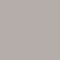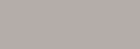# ⚽ ｜ [Tokai Student Soccer League Match 2nd Section 4] Starting soon!Aichi Toho vs Aichi ShukutokuPhoto Soccer Match Summary

### [Tokai Student Soccer League Match 2nd Section 4] Starting soon!Aichi Toho vs Aichi Shukutoku

If you write the contents roughly
After this, from 5/8 19:00, the match between Tokai Student Soccer League Match 2nd Section 4 Aichi Toho University (Men's) vs Aichi Shukutoku University (Men's) will be held at Gifu Kyoritsu University G.

→ Continue readingPlayer!

Sports entertainment application "Player!". We will deliver the latest news, breaking news, schedules, results, etc. of a wide range of sports from soccer, baseball to horse racing to athletics faster than anywhere else.

### Wikipedia related words

If there is no explanation, there is no corresponding item on Wikipedia.# 800

800(Eight hundred, Happy, Yao)Natural number,AlsoIntegerAt799Next to801Is the number before.

## An integer between 801 and 899

### 801 to 820

801 = 32 × 89,Harshad number,Yaoi Ofpun

802 = 2 x 401, 8 consecutive素 数Sum (83 + 89 + 97 + 101 + 103 + 107 + 109 + 113),Non-tortient

803 = 11 x 73, Harshad number, sum of 3 consecutive prime numbers (263 + 269 + 271), sum of 9 consecutive prime numbers (71 + 73 + 79 + 83 + 89 + 97 + 101 + 103 + 107)

804 = 22 × 3 × 67, Harshad number, non-tortient

805 = 5 × 7 × 23,Wedge number

806 = 2 x 13 x 31, sphenic number, nontotient, August 1945, 8HiroshimaMMDD

807 = 3 × 269

808 = 23 × 101,7-segment displayIn the display ofPoint symmetryIt is a number.

809 : Prime number, 31stTwin prime(809, 811),Sophie Germain prime,Prime number, August 1945, 8NagasakiMMDD

810 = 2 × 34 × 5, Harshad number

811 = 53 + 73 + 73, Prime numbers, 181 with swapped numbers is also prime numbers, 31st twin prime numbers (809, 811), Chen prime numbers, sum of 5 consecutive prime numbers (151 + 157 + 163 + 167 + 173),JR Kyushu 811 series

812 = 22 × 7 × 29 = 45 - 35 + 25 - 15 = 28 x 29, number of 28th rectangles

813 = 3 x 271 = 280 + 281 + 282 ,JR Kyushu 813 series

814 = 2 × 11 × 37, wedge number, nontortient

815 = 5 × 163, August 1945, 8HiroshimaHHMM at the time of the atomic bomb explosion,The end of the warMMDD,JR Kyushu 815 series

816 = 24 × 3 × 17, 16thNumber of triangular pyramids, Zuckerman number

817 = 19 x 43, sum of three consecutive prime numbers (3 + 269 + 271),JR Kyushu 817 series

818 = 2 x 409, non-tortient

819 = 32 × 7 × 13 = 93 + 92 + 9 = 45 - 44 + 43 - 42 + 4 − 1, 13thNumber of quadrangular pyramids (819 = 12 + 22 +… + 132),JR Kyushu BEC819 series

820 = 22 × 5 × 41 = 93 + 92 + 9 + 1 = 45 - 44 + 43 - 42 + 4, 40thTriangular number, Harshad number

### 821 to 840

821 : 素 数, 32ndTwin prime(821, 823),Quadruplet prime (821, 823, 827, 829), swapped numbers281Is also a prime number

822 = 2 x 3 x 137, sphenic number, sum of 12 consecutive prime numbers (43 + 47 + 53 + 59 + 61 + 67 + 71 + 73 + 79 + 83 + 89 + 97)

823 : Prime numbers, 32nd twin prime numbers (821, 823), quadruplet prime numbers (821, 823, 827, 829), numbers replaced283Is also a prime number

824 = 23 × 103, sum of 10 consecutive prime numbers (61 + 67 + 71 + 73 + 79 + 83 + 89 + 97 + 101 + 103), nontotient

825 = 3 × 52 × 11, Smith number, Harshad number

826 = 2 × 7 × 59, wedge number

827 : Prime number, 33rd twin prime number (827, 829), quadruplet prime number (821, 823, 827, 829), sum of 7 consecutive prime numbers (103 + 107 + 109 + 113 + 127 + 131 + 137) , Chen prime

828 = 22 × 32 × 23, Harshad number,7-segment displayIn the display ofPoint symmetryIt is a number.

829 : Prime number, 33rd twin prime number (827, 829), quadruplet prime number (821, 823, 827, 829), sum of 3 consecutive prime numbers (271 + 277 + 281), Chen prime number

830 = 2 x 5 x 83, sphenic number, sum of four consecutive prime numbers (4 + 197 + 199 + 211), nontotient

831 = 3 x 277, in chat, etc.SlangMeaning of "I love you" (8)The alphabet, Three単 語, From one meaning).

832 = 26 × 13, Harshad number

833 = 72 × 17

834 = 2 x 3 x 139, sphenic number, sum of 6 consecutive prime numbers (127 + 131 + 137 + 139 + 149 + 151), nontotient

835 = 5 × 167

836 = 22 × 11 × 19, 2thWeird number

837 = 33 × 31

838 = 2 × 419

839 : Prime, safety prime, Chen prime, sum of 5 consecutive primes (157 + 163 + 167 + 173 + 179)

840 = 23 × 3 × 5 × 7. It is the 13th highly composite number and has about 32 divisors. The minimum number divisible by all the numbers 1 to 10 except 9, the Harshad number, the sum of twin primes (419 + 421

### 841 to 860

841 = 292 = 202 + 212 , 29ndSquare number, Sum of 3 consecutive primes (277 + 281 + 283), sum of 9 consecutive primes (73 + 79 + 83 + 89 + 97 + 101 + 103 + 107 + 109), centered square, centered Hexagon number, octagon number with center

842 = 2 x 421, non-tortient

843 = 3 × 281

844 = 22 × 211, non-tortient

845 = 5 × 132

846 = 2 × 32 × 47, sum of 8 consecutive prime numbers (89 + 97 + 101 + 103 + 107 + 109 + 113 + 127), nontortient, Harshad number

847 = 7 × 112

848 = 24 × 53

849 = 3 × 283

850 = 2 × 52 × 17, non-tortient

851 = 23 x 37,FM OsakaAbbreviation of

852 = 22 × 3 × 71, 24thPentagon, Smith number "Taiko no Tatsujin"Practice song op.10-4" by 852 combo.

854 =2 × 7 × 61, wedge number, nontortient

855 = 32 × 5 × 19

856 = 23 ×107, pentagon with center

857 : Prime number, 34stTwin prime(857, 859), sum of three consecutive prime numbers (3 + 281 + 283), Chen prime number, 293 with swapped numbers is also a prime number

858 = 2 × 3 × 11 × 13,Four element composite number, Nippon Professional Baseball (NPB) The total number of games played by the baseball team in the regular season (since 2015),7-segment displayIn the display ofPoint symmetryIt is a number.

859 : Prime number, 34th twin prime number (857, 859)

860 = 22 × 5 × 43, sum of four consecutive prime numbers (4 + 199 + 211 + 223)

### 861 to 880

861 = 3 × 7 × 41, the 41st triangular number, the 21stHexagon, Sphenic number, smith number

862 = 2 × 431

863 : 素 数, Safe prime, Chen prime, sum of 5 consecutive primes (163 + 167 + 173 + 179 + 181), sum of 7 consecutive primes (107 + 109 + 113 + 127 + 131 + 137 + 139), numbers 683 also replaced with a prime number

864 = 25 × 33, The sum of twin primes (431 + 433),Harshad number, The sum of 6 consecutive prime numbers (131 + 137 + 139 + 149 + 151 + 157)

865 = 5 × 173

866 = 2 x 433 = 133 - 113 , Non-tortient

867 = 3 × 172

868 = 22 × 7 × 31, Nontotient,Professional baseballtotalHome runThe highest number record is 868 (Sadaharu Oh

869 = 11 × 79, three consecutive sums of squares (32 + 172 + 182

870 = 2 × 3 × 5 × 29, 29thNumber of rectangles, Harshad number, sum of 10 consecutive prime numbers (67 + 71 + 73 + 79 + 83 + 89 + 97 + 101 + 103 + 107), nontotient

871 = 13 × 67

872 = 23 × 109, non-tortient

873 = 32 × 97 = 1! + 2! + 3! + 4! + 5! + 6!

874 = 2 × 19 × 23, 19thHexagonal number, Wedge number, sum of the first 23 prime numbers, nontortient,19Minimum Harshad number based on

875 = 53 × 7

876 = 22 × 3 × 73

877 : Prime number,Bell number, Chen prime

878 = 2 x 439, non-tortient

879 = 3 × 293

880 = 24 × 5 × 11,Harshad number

### 881 to 899

881 : 素 数, 35ndTwin prime(881, 883), the sum of 9 consecutive prime numbers (79 + 83 + 89 + 97 + 101 + 103 + 107 + 109 + 113),Prime number

882 = 2 × 32 × 72,Harshad number

883 : Prime number, 35th twin prime number (881, 883), sum of 3 consecutive prime numbers (283 + 293 + 307)

884 = 22 × 13 × 17

885 = 3 × 5 × 59,Wedge number

886 = 2 × 443

887 : Prime number,Safety prime, Chen prime

888 = 23 × 3 × 37, sum of eight consecutive prime numbers (8 + 97 + 101 + 103 + 107 + 109 + 113 + 127), Harshad number,7-segment displayIn the display ofPoint symmetryIt is a number.

889 = 127 x 7 = 13 + 23 + 33 + 53 + 63 + 83 = (3 − 1/2)3 + (5 − 1/2)3 + (7 − 1/2)3 + (11 − 1/2)3 + (13 − 1/2)3 + (17 − 1/2)3

890 = 2 x 5 x 89 = 103 - 102 -10, sphenic number, sum of four consecutive prime numbers (4 + 211 + 223 + 227), nontotient

891 = 34 × 11, the sum of 5 consecutive prime numbers (167 + 173 + 179 + 181 + 191)

892 = 22 × 223, non-tortient

893 = 19 x 47,Condiment Ofpun,Yakuza

894 = 2 × 3 × 149, wedge number, nontortient

895 = 5 × 179, Smith number

896 = 27 × 7, the sum of 6 consecutive prime numbers (137 + 139 + 149 + 151 + 157 + 163)

897 = 3 × 13 × 23, wedge number

898 = 2 x 449, non-tortient

899 = 29 × 31

## Related item

An integer between 801 and 899
800801802803804805806807808809
810811812813814815816817818819
820821822823824825826827828829
830831832833834835836837838839
840841842843844845846847848849
850851852853854855856857858859
860861862863864865866867868869
870871872873874875876877878879
880881882883884885886887888889
890891892893894895896897898899

Back to Top
Close